## General Question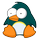# What is the answer to this math problem?

Asked by justanswerthequestion (106) June 16th, 2010

I know the answer, I am just not getting it right?
½x + ½y
THANKS:)

Observing members: 0Composing members: 0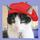There is no equation. Therefore x and y can be whatever you want them to be…pizza slices and spark plugs.

gailcalled (54644)“Great Answer” (4) Flag as…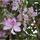½ (x+y) is what I think you are looking for !!!! A simplification to….. .

i.e x= 4 and y=9

½ * 4 + ½ *9 is same as 2+4.5 = 6.5 or

½( 4 + 9 ) or ½ * 13 or 6.5 or any other numbers for x or y.

Tropical_Willie (30481)“Great Answer” (2) Flag as…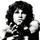Yo yo no homework questions on here

icehky06 (896)“Great Answer” (3) Flag as…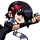This isn’t a problem, it’s just two terms summed together.

Solve A + B. You can’t, there isn’t anything to solve.

Ivan (13479)“Great Answer” (1) Flag as…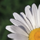@icehky06 :: They are allowed now. You just can’t give the answer away. But you can help explain how to find the answer.

jrpowell (40552)“Great Answer” (2) Flag as…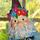The answer to what? As others already said, there is no problem here as stated. What are you trying to solve for?

lilikoi (10105)“Great Answer” (1) Flag as…ok then the text book is wrong

justanswerthequestion (106)“Great Answer” (1) Flag as…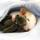Post the answer from the textbook.

ratboy (15167)“Great Answer” (1) Flag as…i dont know because it only gives odd numbers and this one is even

justanswerthequestion (106)“Great Answer” (1) Flag as…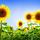Show us a similar problem from the odd ones and give us the answer.

JLeslie (64656)“Great Answer” (0) Flag as…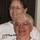@justanswerthequestion Please explain how the textbook is wrong if there is no answer shown? P.S. It is no uncommon for errors to occur in textbooks.

Without an = there is no equation. I’m pretty sure what they are looking for is simplifying the expression or explaining it.

You explain a problem by randomly assigning the x and the y and then see if it works.

If you assign 2 to x and 4 to y you can explain the expression this way: ½ of 2 + ½ of 4 is the same as ½ of 2 + 4. Then work it out to make sure it agrees.

YARNLADY (46142)“Great Answer” (0) Flag as…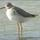I miss algebra class… Wait no I don’t.

mowens (8403)“Great Answer” (1) Flag as…@justanswerthequestion You said “I know the answer”. Well what is it then? Makes no sense you are asking us for the answer if you already know it. What was the question? You’re giving us a mathematical phrase. If you would tell us what the question was and what you mean by “I know the answer” maybe we could get somewhere here.

lilikoi (10105)“Great Answer” (1) Flag as…or